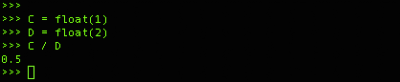## Friday, May 01, 2009

### int and float variables in PythonWhen you start working and coding with python and this is your first experience with coding you will lost likely be puzzeld at first. Playing with numbers in coding can sometimes be somewhat strange because we can create different type of variables for numbers. manly you will be using FLOAT and INT values. where float numbers contain decimals int values will not.

some examples, lets first define some variables. We will create a variable A which will have the value 1 and we will create a variable B which will hold the value 2. When the are created we will divide A by B which by all common sense will give you 0.5 however as you can see in the example below it will give you 0 as the result instead of 0.5The reason for this is that we have defined variables A and B as int values, we did not explicitly defined them as int however based upon the content of the variable it is defined as a int and a int value is not able to hold decimals. Because Python is now thinking we are working with only int values the answer is also a int value. We can define variables explicitly as a type of variable, for example if we wanted to have them represented as float values we define this with float(). Notice the example below.Now the result will also be a float value and it will state 0.5 instead of 0. However if you do not want to define your variables explicitly as a float you can always "change" this when you do a operation on them. As you can see in the example below we have a variable a and b and they are int values as we can see at the outcome of dividing a by b. However now we do the same calculations and now we state that variable b is a float and the answer is suddenly a answer in float format. however, this is only limited to the operation as you can see because if we divide a by b again it is again a int value.You can change the type of variable of variables a and b permanently, you can state that a = float(a) and then you will have them changed (until you change them to something else) You can see a example of it below.Now you can come to a point that you are no longer sure what your variable is exactly, the type() function can help you with this. Just use the type() function in combination with your variable and you will be presented with the type of variable.